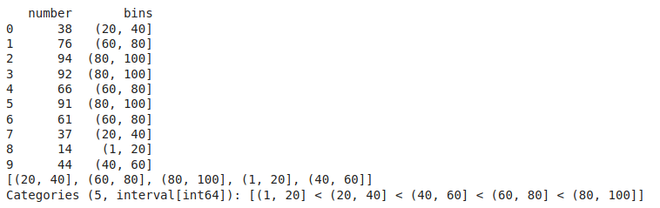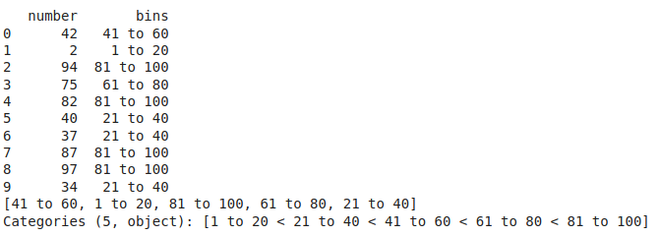# Pandas.cut() method in Python

Pandas cut() function is used to separate the array elements into different bins . The cut function is mainly used to perform statistical analysis on scalar data.

Syntax: cut(x, bins, right=True, labels=None, retbins=False, precision=3, include_lowest=False, duplicates=”raise”,)

Parameters:

x: The input array to be binned. Must be 1-dimensional.

bins: defines the bin edges for the segmentation.

right : (bool, default True )  Indicates whether bins includes the rightmost edge or not. If right == True (the default), then the bins [1, 2, 3, 4] indicate (1,2], (2,3], (3,4].

labels : (array or bool, optional)  Specifies the labels for the returned bins. Must be the same length as the resulting bins. If False, returns only integer indicators of the bins.

retbins : (bool, default False) Whether to return the bins or not. Useful when bins is provided as a scalar.

Exmaple 1: Let’s say we have an array of 10 random numbers from 1 to 100 and we wish to separate data into 5 bins of (1,20] , (20,40] , (40,60] ,  (60,80] , (80,100] .

## Python3

 `import` `pandas as pd ` `import` `numpy as np ` ` `  ` `  `df``=` `pd.DataFrame({``'number'``: np.random.randint(``1``, ``100``, ``10``)}) ` `df[``'bins'``] ``=` `pd.cut(x``=``df[``'number'``], bins``=``[``1``, ``20``, ``40``, ``60``,  ` `                                          ``80``, ``100``]) ` `print``(df) ` ` `  `# We can check the frequency of each bin ` `print``(df[``'bins'``].unique())`

Output:Example 2: We can also add labels to our bins, for example let’s look at the previous example and  add some labels to it

## Python3

 `import` `pandas as pd ` `import` `numpy as np ` ` `  `df ``=` `pd.DataFrame({``'number'``: np.random.randint(``1``, ``100``, ``10``)}) ` `df[``'bins'``] ``=` `pd.cut(x``=``df[``'number'``], bins``=``[``1``, ``20``, ``40``, ``60``, ``80``, ``100``], ` `                    ``labels``=``[``'1 to 20'``, ``'21 to 40'``, ``'41 to 60'``, ` `                            ``'61 to 80'``, ``'81 to 100'``]) ` ` `  `print``(df) ` ` `  `# We can check the frequency of each bin ` `print``(df[``'bins'``].unique()) `

Output:My Personal Notes arrow_drop_upCheck out this Author's contributed articles.

If you like GeeksforGeeks and would like to contribute, you can also write an article using contribute.geeksforgeeks.org or mail your article to contribute@geeksforgeeks.org. See your article appearing on the GeeksforGeeks main page and help other Geeks.

Please Improve this article if you find anything incorrect by clicking on the "Improve Article" button below.

Article Tags :

Be the First to upvote.

Please write to us at contribute@geeksforgeeks.org to report any issue with the above content.